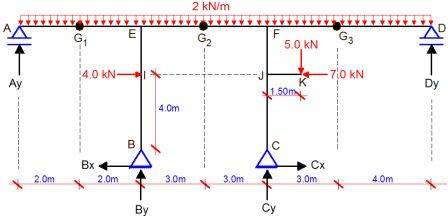# Analysis of Statically Determinate Frames (1)In the paper downloadable in this post, the frame loaded as shown above was completely analysed using the equation of statics to obtain the support reactions, bending moment diagram, shear force diagram, and axial force diagram. See the results below.

Support reactions

Bending moment diagram

Shear force diagram

Axial force diagram